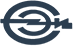# A novel numerical optimality technique to find the optimal results of Volterra integral equation of the second kind with discontinuous kernel

Статья в журнале
Noeiaghdam S., Sidorov D., Dreglea A.
2023
In this study we consider linear and nonlinear Volterra integral equations (VIEs) of the second kind with discontinuous kernel. A novel iterative method using floating point arithmetic (FPA) is presented to solve the problem. Also a convergence theorem and error analysis of the method are presented. The main novelty of this study is to validate the results using the CESTAC (Controle et Estimation Stochastique des Arrondis de Calculs) method which is based on stochastic arithmetic (SA). Moreover instead of applying usual mathematical softwares we use the CADNA (Control of Accuracy and Debugging for Numerical Applications) library which must be done on Linux operating system using C, C++ or Fortran codes. Applying the method and the library we will be able to find the optimal results such as optimal error, optimal iteration of the method and optimal approximation. Proving the main theorem of the CESTAC method we show the equality between the number of common significant digits (NCSDs) of two successive iterations with the exact and approximate solutions. Thus it can help us to apply a new termination criterion instead of absolute error to show the accuracy and efficiency of the method. © 2023 IMACS

### Библиографическая ссылка

Noeiaghdam S., Sidorov D., Dreglea A. A novel numerical optimality technique to find the optimal results of Volterra integral equation of the second kind with discontinuous kernel // Applied Numerical Mathematics. Vol.186. 2023. P.202-212. DOI: 10.1016/j.apnum.2023.01.011
SCOPUS
x
x